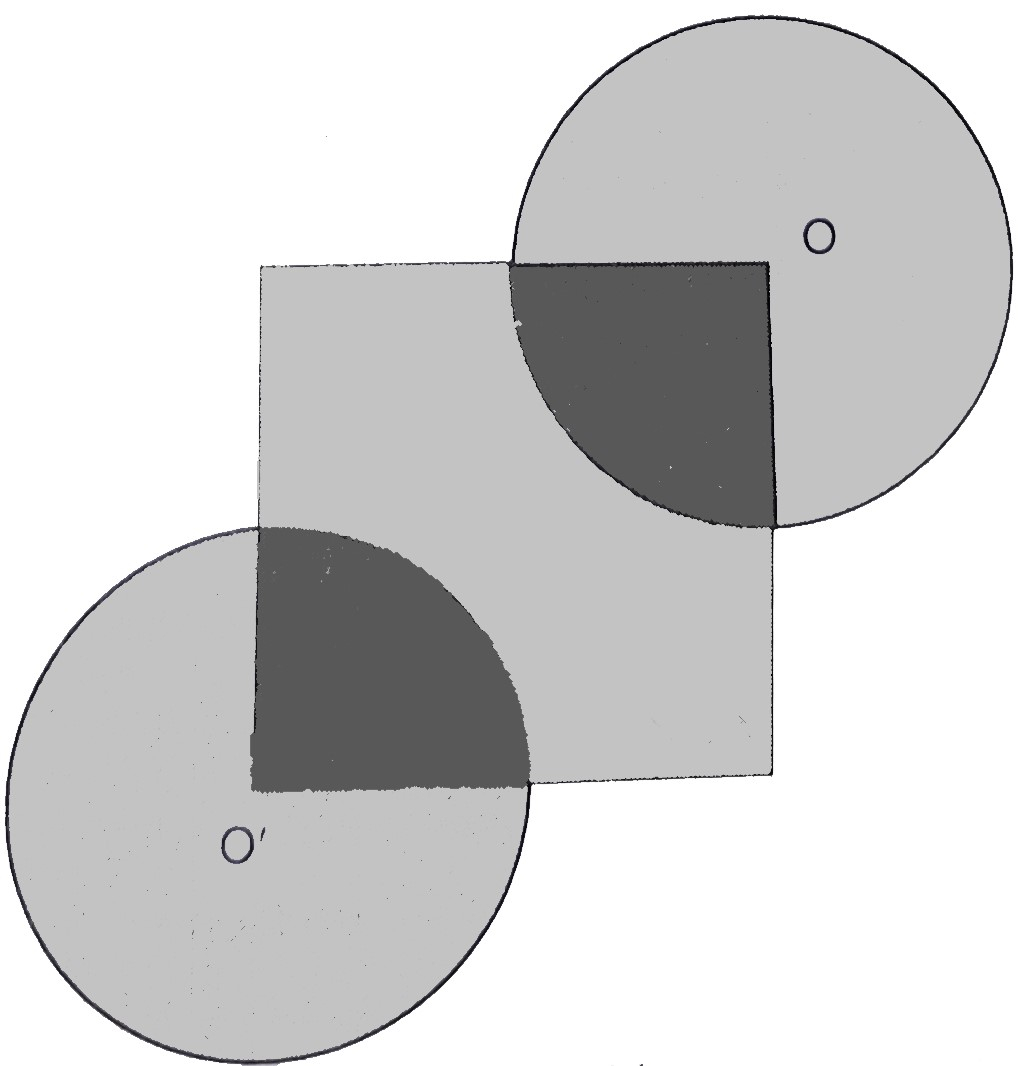"
">

# In the given figure, the side of square is $28 \mathrm{~cm}$, and radius of each circle is half of the length of the side of the square where $O$ and $O^{\prime}$ are centres of the circles. Find the area of shaded region."

Given:

The side of square is $28 \mathrm{~cm}$, and radius of each circle is half of the length of the side of the square where $O$ and $O^{\prime}$ are centres of the circles.

To do:

We have to find the area of the shaded region.

Solution:

Side of the square $=28 \mathrm{~cm}$

Radius of the circle $=\frac{28}{2}$

$=14 \mathrm{~cm}$

Therefore,

Area of the shaded region $=$ Area of square $+2\times\frac{3}{4}$ Area of circle

$=$ Area of square $+\frac{3}{2}$ Area of circle

$=(28)^{2}+\frac{3}{2} \times \frac{22}{7} \times 14^2$

$=784+924$

$=1708 \mathrm{~cm}^{2}$

The area of shaded region is $1708 \mathrm{~cm}^{2}$.

Updated on: 10-Oct-2022

73 Views